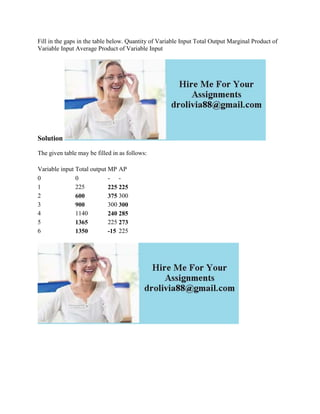Successfully reported this slideshow.

# Fill in the gaps in the table below- Quantity of Variable Input Total.docx×

# Fill in the gaps in the table below- Quantity of Variable Input Total.docx

Fill in the gaps in the table below. Quantity of Variable Input Total Output Marginal Product of Variable Input Average Product of Variable Input
Solution
The given table may be filled in as follows:

.

Fill in the gaps in the table below. Quantity of Variable Input Total Output Marginal Product of Variable Input Average Product of Variable Input
Solution
The given table may be filled in as follows:

.